Next: Measurement of the Luminosity Up: Luminosity Spectrum Previous: Luminosity Spectrum

## Event generation

In the past, an empirical formula used to describe the distribution of the collision energy at next-generation linear colliders has been presented by Yokoya and Chen. In addition, recently developed software, called CAIN, is capable of simulating detailed beam-beam interactions at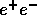,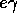and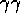linear colliders. The function in  has been found to give a good approximation to the CAIN calculation, as shown in Figure 14.17 at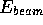=250GeV. Table 14.1 summarizes the relevant parameters for the calculations.

Figure 14.17 shows that the function reasonably reproduce the CAIN result at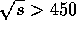GeV, where a small difference seen in the figure is due to a beam disruption included in the CAIN.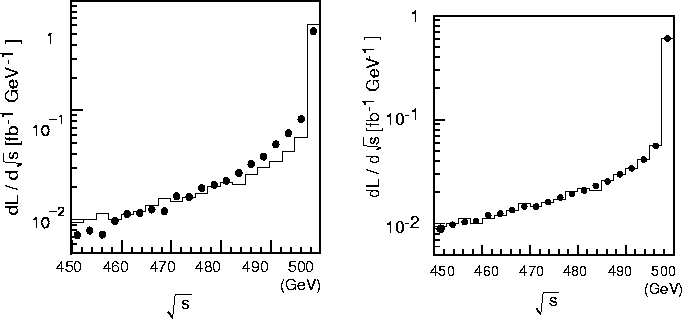Figure 14.18: Luminosity spectra calculated by the generator (histogram) and the function (solid circles).
Figure 14.17: Luminosity spectra calculated by the empirical function (histogram) and CAIN (solid circles).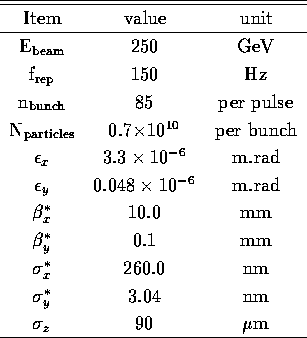Table 14.1: Major parameters of JLC.

To improve the efficiency of calculations, a luminosity-spectrum generator code has been created. The generator code precisely reproduces the empirical function as shown in Figure 14.18.

It should be noted that besides the beam-beam interactions, the beam-energy spread also affects the luminosity-spectrum. Its effects were simulated, and the results are shown in Figure 14.19. In these calculations a uniform energy spread of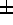1% was assumed for the original beams. It can be seen that the luminosity peaks are smeared at around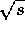=500GeV.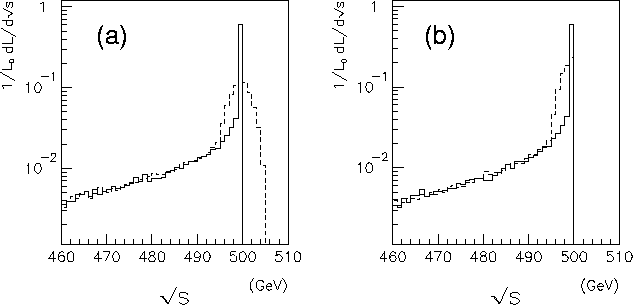Figure 14.19: (a) The luminosity-spectra weighted by the Bhabha cross section, without (with) the1% uniform beam-energy spread, which is shown by the solid (dashed) histogram. (b) The spectra calculated by the acollinearity angles of the Bhabha events, where no entry above=500GeV exists by definition.

The differential cross section of the Bhabha scattering process was calculated using the program GRACE. This is a program package for automatic amplitude calculations. The numerical integration and unweighted event generation were performed by two programs, BASES and SPRING, respectively. In this study, the lowest order Feynman diagrams of this process were taken into account, since the effect of the initial state radiation can be precisely estimated.Next: Measurement of the Luminosity Up: Luminosity Spectrum Previous: Luminosity Spectrum

Toshiaki Tauchi
Thu, May 29, 1997 04:47:48 PM